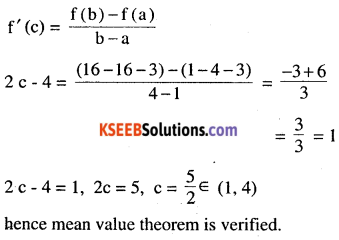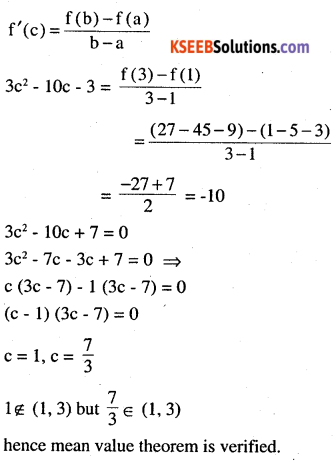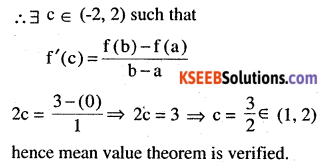# 2nd PUC Maths Question Bank Chapter 5 Continuity and Differentiability Ex 5.8

Students can Download Maths Chapter 5 Continuity and Differentiability Ex 5.8 Questions and Answers, Notes Pdf, 2nd PUC Maths Question Bank with Answers helps you to revise the complete Karnataka State Board Syllabus and score more marks in your examinations.

## Karnataka 2nd PUC Maths Question Bank Chapter 5 Continuity and Differentiability Ex 5.8

### 2nd PUC Maths Continuity and Differentiability NCERT Text Book Questions and Answers Ex 5.8

Question 1.
Verify Rolle’s theorem for the function f (x) = x2 + 2x – 8, x ∈ [-4, 2].
f (x) = x2 + 2x – 8, being polynomial function it is continuous on [-4,2]
Also f’ (x) = 2x + 2
hence derivable on (-4, 2)
f (-4) = (-4)2 + 2 (-4) -8 = 0
f (2) = (2)2 + 2 (2) – 8 = 0
hence f (a) = f (b) i.e. f (-4) = f (2)
∴ All the three conditions of Rolle’s theorem are verified
∴ at least one value c e (-4, 2) such that f’ (c) = 0
f'(c) = 2c + 2, f’c = 0
⇒ c = -I ∈ (-4,2)
hence Rolle’s theorem is verified.Question 2.
Examine if Rolle’s theorem is applicable to any of the following functions. Can you say some thing about the converse of Rolle’s theorem from these example ?

(i) f (x) = [x] for x ∈ [5, 9]
f (x) = |x] being greatest integer function it is not differentiable at integral points.
Hence not continuous on integral points
∴  f (x) is not continuous [5, 9]
Rolle’s theorem is not applicable to f (x) in [5,9]
How ever f’ (x) = 0 ∀ non integral values in [5, 9] thus converse of Rolle’s theorem is not true.

(ii) f (x) = [x] for x ∈ [-2, 2]
The solution is same above only the in­tervals changed.

(iii) f (x) = x2 -1 for x ∈ [1, 2]
f (x) = x2 – 1
being polynomial function f (x) is differentiable ∀ x ∈ [1, 2]
∴ f (x) is continuous is [1, 2]
Also f’ (x) exist, f (x) is derivable on (1, 2)
f (1) = (1)2 – 1 = 0, f (2) = (2)2 – 1 =3
f(1) ≠ f(2)
Since all the conditions are not satisfied, the Rolle’s theorem is not applicable in the given intervals.
Thus the converse of Rolle’s theorem does not hold. That is if the condition of Rolle’s theorem are not satisfied by a function f (x) on some interval [a, b] then f’ (x) may or may not be zero in some point in (a, b).

Question 3.
If f : [-5, 5] → R is a differentiable function and if f’ (x) does not vanish anywhere, then prove that f (-5) ≠ f (5).
It we suppose that f (-5) = f (5), then f would satisfy all the conditions of Rolle’s
theorem on [-5,5] as differentiable P1 is always con­tinuous. Hence there would exist at least one c ∈ (-5,5) such that f’ (c) = 0. Given that f’ (x) does not vanish anywhere, therefore our as­sumption is wrong, hence f (-5) ≠ f (5)Question 4.
Verify Mean Value Theorem, if f (x) = x2 – 4x – 3 in the interval [a, b], where a = 1 and
b = 4.
f(x) = x2 – 4x – 3
Being polynomial function! (x) is continuous is {1,4} and f’ (x) = 2x – 4 and
hence differentiable (1,4)
Conditions of Rolle’s theorems are satisfied
∴ 3 a value c ∈ (1,4) such thatQuestion 5.
Verify Mean Value Theorem, if f (x) = x3 – 5x2 – 3x in the interval [a, b], where a = 1 and b = 3. Find all c ∈ (1, 3) for which f’ (c) = 0.
Being polynomial function f (x) is con­tinuous in (1,3) and diff. in (1, 3).
∴ conditions of mean value theorem are verified.
∴ ∃ a value c ∈ (1, 3) such thatQuestion 6.
Examine the applicability of Mean Value Theorem for all three functions given in the above exercise 2.